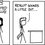# Polynomials? That sounds familiar!

Another CMI problem: (and I could solve this)

Define polynomials $$P_n(x)$$ in this way:

1. $\displaystyle P_0(x) = 1$

2. $\displaystyle P_n(x) = \dfrac{x(x-1)(x-2)\ldots(x-n)} {n!}$ where $n$ is a positive integer.

Prove that for any polynomial $f(x)$, there always exist unique real numbers $b_0, b_1, \ldots b_n$ such that:

$\displaystyle f(x) = \sum_{0 \le i \le n} b_i p_i (x)$

Further prove that the $b_i$'s are all integers, if it is given that $f(m)$ is an integer for each integer $m$.Note by Parth Thakkar
7 years, 2 months ago

This discussion board is a place to discuss our Daily Challenges and the math and science related to those challenges. Explanations are more than just a solution — they should explain the steps and thinking strategies that you used to obtain the solution. Comments should further the discussion of math and science.

When posting on Brilliant:

• Use the emojis to react to an explanation, whether you're congratulating a job well done , or just really confused .
• Ask specific questions about the challenge or the steps in somebody's explanation. Well-posed questions can add a lot to the discussion, but posting "I don't understand!" doesn't help anyone.
• Try to contribute something new to the discussion, whether it is an extension, generalization or other idea related to the challenge.

MarkdownAppears as
*italics* or _italics_ italics
**bold** or __bold__ bold
- bulleted- list
• bulleted
• list
1. numbered2. list
1. numbered
2. list
Note: you must add a full line of space before and after lists for them to show up correctly
paragraph 1paragraph 2

paragraph 1

paragraph 2

[example link](https://brilliant.org)example link
> This is a quote
This is a quote
    # I indented these lines
# 4 spaces, and now they show
# up as a code block.

print "hello world"
# I indented these lines
# 4 spaces, and now they show
# up as a code block.

print "hello world"
MathAppears as
Remember to wrap math in $$ ... $$ or $ ... $ to ensure proper formatting.
2 \times 3 $2 \times 3$
2^{34} $2^{34}$
a_{i-1} $a_{i-1}$
\frac{2}{3} $\frac{2}{3}$
\sqrt{2} $\sqrt{2}$
\sum_{i=1}^3 $\sum_{i=1}^3$
\sin \theta $\sin \theta$
\boxed{123} $\boxed{123}$

Sort by:

Other problems I found interesting:

- 7 years, 2 months ago

did any one get a seat in CMI (i got one wihtout interview) i solved nearly 6 of the ques....

- 7 years ago

I did (without interview). Didn't expect it though! Pretty much set on joining there.

- 7 years ago

Part (a): Proving the existence and uniqueness of ${b_i}$:

Let $f(x)= c_0 +c_1x +c_2x^2+...+c_nx^n$ and take note of the fact that:

$p_n(x)= \dbinom{x}{n}(x-n)$. Since $p_0(x)=1$, we may write:

$\sum_{0 \le i \le n}(b_i)(p_i(x)) = (b_0)+ \sum_{1 \le i \le n}(b_i)(p_i(x))$

Expanding $\sum_{1 \le i \le n}(b_i)(p_i(x))$: (Without loss of generality, assume $\sum_{1 \le i \le n}(b_i)(p_i(x))$= $\sum_{i=1}^{n}(b_i)(p_i(x))$)

$\sum_{i=1}^{n}(b_i)(p_i(x))$)= $b_1(x^2-x)+\frac{b_2}{2}(x^3-3x^2+2x)+\frac{b_3}{6}(x^4-6x^3+11x^2-6x)+\frac{b_4}{24}(x^5-10x^4+35x^3-50x^2+24x)+... +\frac{b_nx!(x-n)}{n!(x-n)!}$

Equating coefficients of f(x), $c_i$, with those of $\sum_{i=1}^{n}(b_i)(p_i(x))$):

$c_0=b_0$

$c_1=-b_1+b_2-b_3+b_4+....=\sum_{i=1}^{n}(-1)^ib_i$

$c_2=b_1-\frac{3}{2}b_2+\frac{11}{6}b_3-\frac{50}{24}b_4+....$

$c_3= \frac{b_2}{2} - b_3 +\frac{35}{24}b_4-....$

$c_4= \frac{b_3}{6} - \frac{10}{24}b_4+.....$

$c_5= \frac{b_4}{24}+....$

This is a linear system of n equations (excluding $c_0=b_0$) in n unknowns. Therefore, we may construct an n x n matrix A such that: $A\vec{b}=\vec{c}$, where $\vec{b}$ contains all $b_i$ and $\vec{c}$ contains all $c_i$. $A=$

$\begin{pmatrix} -1 & 1 & -1&...&\alpha_1 \\ 1 & \frac{-3}{2} & \frac{11}{6}&...&\alpha_2 \\ 0 & \frac{1}{2} & -1&...&\alpha_3\\ 0&0&\frac{1}{6}&...&\alpha_4\\ .&.&.&.&.\\ .&.&.&.&.\\ .&.&.&.&.\\ 0&0&0&....&\alpha_n\\ \end{pmatrix}$

$A\vec{b}=\vec{c}$ has a unique solution if and only if A is an invertible matrix. A is invertible if and only if $det(A)\neq 0$. Then $A\vec{b}=\vec{c}$ has a unique solution if and only if $det(A)\neq 0$. If we use Gaussian Elimination to reduce A, that is, if we add line (1) to line (2), then line (2) to line (3), then line (3) to line (4), etc. we see that A will be reduced to an upper triangular matrix with every term on the main diagonal of A of the form: $A_{ji}=\frac{-1}{i!}$, where i corresponds to the column number of $A_{ji}$. Hence $det(A)=\prod_{i=1}^n\frac{-1}{i!}$.

Since $det(A)=\prod_{i=1}^n\frac{-1}{i!}$ and $i\ge1$, $det(A)\neq 0$. Then A is invertible and $A\vec{b}=\vec{c}$ has a unique solution, hence $\vec{b}=A^{-1}\vec{c}$, which of course exists. This proves the existence of $b_i$ but does not prove the uniqueness of all $b_i$. We will now proceed to prove this.

Let $A_{ji}^{-1}$ denote the element of $A^{-1}$ in the $j^{th}$ row and $i^{th}$ column. Since $\vec{b}=A^{-1}\vec{c}$, we have:

$b_n=A_{n1}^{-1}c_1 + A_{n2}^{-1}c_2+A_{n3}^{-1}c_3+...+A_{nn}^{-1}c_n$

Suppose $\exists$ $b_i$ such that $b_i=b_n$ but $i \neq n$, i.e. suppose all $b_i$ are not unique. Then, since $b_n$ is described by the above equation:

$A_{i1}^{-1}c_1=A_{n1}^{-1}c_1$

$A_{i2}^{-1}c_2=A_{n2}^{-1}c_2$

$A_{i3}^{-1}c_3=A_{n3}^{-1}c_3$

And in general: $A_{in}^{-1}c_n=A_{nn}^{-1}c_n$ $\Rightarrow$ $A_{in}^{-1}=A_{nn}^{-1}$

If $A_{in}^{-1}=A_{nn}^{-1}$ and $i \neq n$, then $A^{-1}$ contains at least two identical rows, which implies $det(A^{-1})=0$. Then $A^{-1}$ is not invertible, which is impossible because $(A^{-1})^{-1}=A$. This is a contradiction, hence we are forced to conclude that all $b_i$ are unique. Part (b) coming soon.

QED

- 6 years, 11 months ago

Note that the polynomials $P_i(x), 0\leq i \leq n$ have increasing degrees and hence they are linearly independent in the vector space of polynomials of degree $n$. Hence they form a basis and the proof for the first part follows.

- 6 years, 11 months ago

Solved this one. Nice question.

- 7 years, 2 months ago

How do you solve this question? Can you give the solution? Or atleast an hint

- 7 years, 2 months ago

Hint: Notice that $P_k (x)$ vanishes at $x = k$, and also do the "higher" $P$'s. That is, $P_{k+1}, P_{k+2}$ and so on. But the "lower" $P$'s don't. If you use this properly, you're done!

- 7 years, 2 months ago

Nice :)

- 7 years, 2 months ago